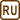## Interference of White Light

### Task number: 1972

Two coherent rays of white light interfere with a path difference of 2.5 μm. Light components of which wavelenths (colors) exhibit an interference maximum?

• #### Hint 1

What is the condition for two coherent waves to exhibit an interference maximum with respect to the path difference between them?

• #### Hint 2

What is the range of wavelengths of the visible light?

• #### Notation

 Δl = 2,5  µm = 2,5·10−6 m The path difference between the two rays λmin = 380 nm = 3,8·10−7 m The minimum wavelength of the visible light λmax = 740 nm = 7,4·10−7 m The maximum wavelength of the visible light λ = ? (nm) The wavelength at which occurs an interference maximum
• #### Solution

Our aim is to calculate the wavelengths of the components of the visible light that produce an interference maximum. This occurs when the path difference Δl between the two rays is an integer product of the wavelength λ. We write this as the following condition:

$\mathrm{\Delta} l = k\lambda,$

where k is the order of the maximum.

We express the searched wavelength λ:

$\lambda = \frac{\mathrm{\Delta}l}{k}. \tag{1}$

To proceed, we need to know the values of k with which the wavelength in Equation 1 falls into the range of visible light.

We express k from the previous formula:

$k = \frac{\mathrm{\Delta}l}{\lambda}.$

And we use the limit values of the visible range of wavelengths:

We input the minimal visible wavelength λmin = 3.80·10−7 m:

$k_{min} \,\dot{=} \frac{2.5 {\cdot} 10^{−6}}{3.80 {\cdot} 10^{−7}} = 6.5.$

And for the maximal visible wavelength λmax = 7,40·10−7 m we get:

$k_{max} \,\dot{=} \frac{2.5 {\cdot} 10^{−6}}{7.40 {\cdot} 10^{−7}} = 3.3.$

An interference maximum occurs for integer multiples k of the wavelenghts. We take the integers that lay in the interval from 3.3 to 6.5. These are k = 4, k = 5, k = 6.

And we use these values of k in equation (1):

 k λ “color” of light 4 625 nm red-orange 5 500 nm blue-green 6 417 nm violet-blue